×#### Thank you for registering.

One of our academic counsellors will contact you within 1 working day.

Click to Chat

1800-1023-196

+91-120-4616500

CART 0

• 0

MY CART (5)

Use Coupon: CART20 and get 20% off on all online Study Material

ITEM
DETAILS
MRP
DISCOUNT
FINAL PRICE
Total Price: Rs.

There are no items in this cart.
Continue Shopping```Limits & Continuity

Table of Content

Limit of a Function

Continuity of a Function

Important formulas of Limit and Continuity

Algebra of Limits

Some Important Limits

L’Hospital’s Rule

Squeeze / Sandwich Theorem

Some Interesting Facts

Is limit and continuity an important part of IIT – JEE preparation?

What are the best books for the preparation of limit and continuity?

Tips to study Limit and Continuity for IIT JEE

Practical Applications of Limit and Continuity

Related ResourcesThe concept of limit and continuity is the backbone of calculus. Each and every notion of calculus can be considered to be a limit in one sense or the other. The concept of limits has also resulted in various other branches of calculus.Continuity is completely dependent on limit. The two concepts go hand in hand.

Limits and continuity is one of the most important topics of various competitive exams like the IIT JEE. Besides being simple, this topic is scoring too. Hence, those eyeing exams like IIT JEE must master this topic in order to remain competitive in the JEE.We shall throw some light on the concepts related to limits and continuity and also discuss various tips to master this topic.

Limit of a Function

A limit is basically a number attained by a function when the independent variable of the function approaches a given value. For example, if we have the function f(x) = 6x, then it is stated as, “the limit of the function f(x) as x approaches 2 is 12. Mathematically, it is expressed as limx→2f(x) = 12.

Continuity of a Function

A function f is said to be continuous at the point x = a if the following conditions are true:

f(a) is defined

limx→ a f(x) exists

limx→a f(x) = f(a).

Both side limits must also be equal i.e. limx→a- f(x) = f (a) = limx→a+ f(x).

When we talk of continuity, another concept that is associated with it is discontinuity of a function. We shall not delve into the intricacies of discontinuity of a function but it is clear from the term itself that a function which is not continuous is said to be discontinuous. The various types of discontinuities have been represented in the form of a chart given below: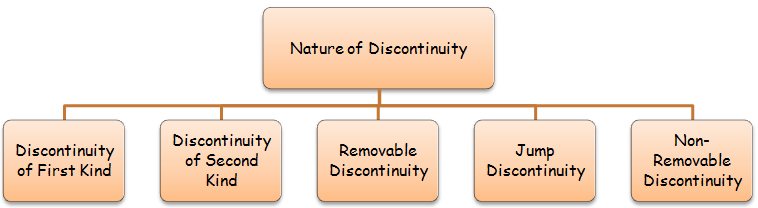Those willing to go into the intricacies of these topics can refer the study material on limit and continuity.

Important formulas of Limit and Continuity

The left hand limit of f(x) as x tends to c is defined to be ‘L’ if as x approaches c from the left, f(x) approaches L. Mathematically, it is represented as

?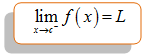The right hand limit of f(x) as x tends to c is defined to be ‘L’ if, as x approaches c from the right, f(x) approaches L. Mathematically, it is represented as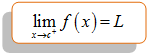?

If the functions f and g are continuous at c, then

f + g is continuous at c

f – g is continuous at c

af is continuous at c for each real a

f.g is continuous at c

f/g is continuous at c provided g(c) ≠ 0

Algebra of Limits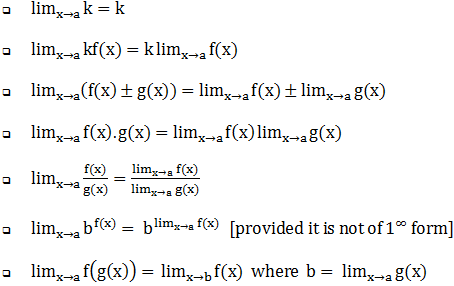Some Important Limits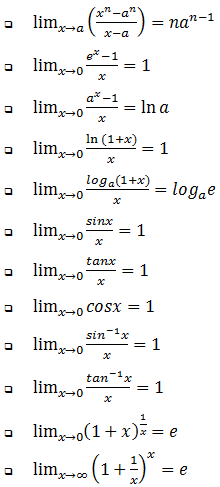L’Hospital’s Rule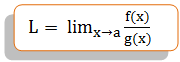If f(x) / g(x) is of 0/0 from, thenSqueeze / Sandwich Theorem

If h(x) < g(x) < f(x)

and Lim x → a h(x) = Lim x → a f(x) = L

then, Lim x → a g(x) = LSome Interesting Facts

The existence of limit of a function at a point does not guarantee the continuity of the function at that particular point

A function is said tobe continuous provided it is continuous at all the points of the domain.

If a function is continuous at some point, then the limit necessarily exists at that point.

The sum, difference, product and quotient(as long as denominator is non-zero) of two continuous functions is continuous.

A function fcontinuous on a closed interval [a, b] attains every value between f(a) and f(b) at least once on the open interval (a, b).

Functions which can be drawn on appear without lifting of pen in between are said to be continuous.

While polynomial functions are always continuous,rational functions are continuous only if the denominator is not equal to zero.

When the left hand limit and the right hand limit at the point are not equal, then we have ‘finite irremovable discontinuity’ at the point.

In case the limit of function exists at a point but either the function is not defined at that point or is defined wrongly, then the function is said to exhibit removable discontinuity.

Limit of sum is the sum of limits.

Integral part of x([x]) has jump discontinuity at every integral point.

Continuity of a function at a particular point does not guarantee its differentiability at that particular point.

A function continuous on a closed interval [a, b] is necessarily bounded if both a and b are finite. This is not true in case of open interval.

If the function u = f(x) is continuous at the point x = a, and the function y = g(u) is continuous at the point u = f(a), then the composite function y = (gof)(x) = g(f(x)) is continuous at the point x = a.

Is limit and continuity an important part of IIT – JEE preparation?

Limit and continuity is an important component of differential calculus which carries weightage of around 15-20% in various competitive exams like the JEE. This chapter also lays the foundation for various other topics of calculus.

What are the best books for the preparation of limit and continuity?

Students are advised not to consult too many books as referring too many texts can lead to confusion. Hence,those eyeing various elite exams like the JEE must refer standard books like:

I.A. Maron

Arihant

Tata McGraw Hill (TMH)

Tips to study Limit and Continuity for IIT JEEFocus on clearing basics and conceptual knowledge rather than solving questions for reaching at the solutions.

Maxima & Minima is a simple topic but consistent practice is essential in order to excel in it.

Practice is the only key to success. Try solving questions using different methods like standard expressions, L’Hospital’s rule, series expansion etc.

Whenever you are about to attempt a question on maxima or minima, read the question carefully to identify what it actually demands.

A problem on limits must first be analyzed using series expansion of the function.

All the important limits and expansions must be on your fingertips.

While solving problems involving limits of integral part functions, try adopting the basic root.

The questions on one-sided limits and continuity should preferably be calculated using the basic definition.

Practice solving questions using L’Hospital’s Rule as it saves time.

Attempting good number of questions from the previous year papers and sample papers is a must.

Practical Applications of Limit and Continuity

Calculus is an extremely important branch of mathematics. This branch is extensively used in various other domains like classical mechanics and electromagnetism. Various concepts like Newton’s laws of motion, moment of inertia etc. are based on the logics of calculus. Some of the chief real life applications include:

A real life example of a continuous function is represented by the temperature throughout the day.

The concept of limits is used in the measurement of instantaneous speed of the vehicles like motorcycle, car, truck etc.

The concept of limit proves helpful in calculating the area of complex geometrical shapes where basic geometry fails.

Another important application of limits and continuity is in the fields of astronomy and time travel.

The interaction between the various species within the eco-system is also studied with the help of concepts of calculus.

Engineers use calculus for building skyscrapers, bridges, robotics etc.

Electrical and computer scientists use this concept for designing various systems.

The earth’s ability of maintaining and sustaining various species is also a real life example of limits.As the limit is approached, or with the end of resources, it eventually reaches zero and the population which needs it, dies.

Calculus is used in modeling engineering problems which are often stated as ordinary or partial differential equations.

Related Resources

To read more, Buy study materials of Limtis and Continuity comprising study notes, revision notes, video lectures, previous year solved questions etc. Also browse for more study materials on Mathematics here.
```### Course Features

• 731 Video Lectures
• Revision Notes
• Previous Year Papers
• Mind Map
• Study Planner
• NCERT Solutions
• Discussion Forum
• Test paper with Video Solution